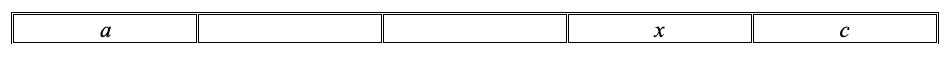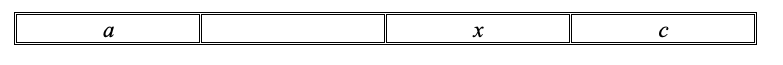# Week 4: Decidedly Average

In the following grids, each blank square should be filled with the mean of the two numbers in adjacent squares.

(a)        Fill in the grid below.(b)       Find x in the following grid, leaving your answer in terms of a,c:(c)        Find x in the following grid, leaving your answer in terms of a,c:(d)       For a grid of length n with first box a and last box c, make a hypothesis for the value of x, the box adjacent to c in terms of a,c,n.(e)        Check your hypothesis works when a = 10, b = 15, n = 6.

Teacher notes:

Level of difficulty:  Accessible for good SL and HL students

Syllabus knowledge required:  Basic averages and algebraic manipulation

This could be done when doing mean, median mode.

Solutions: